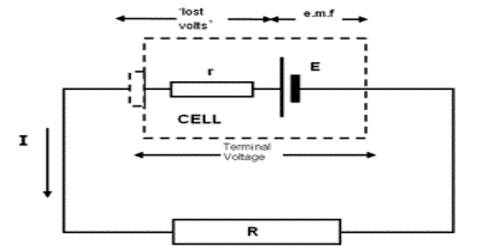Physics

# Lost VoltLost volt: The potential difference between the two terminals of a cell decrease by an amount that does not contribute to the circuit, is called lost volt.

For realistic intentions, the e.m.f. of a cell may be calculated to a very close estimate by taking the reading of a high-resistance voltmeter connected straight across the cell terminals when the cell is not connected to anything else. The cause why this value is only approximate will be explained at the end of this section, but for the time being, we shall ignore the slight fault. Let us suppose that a voltmeter connected to the terminals of a dry cell of internal resistance 2.0 n gives a reading of 1.5 V. This is the e.m.f. of the cell (Figure (a)).A 3.0 n resistor is now connected to the terminals of the cell so that the cell drives a current through it. It is noticed that the voltmeter reading has dropped to 0.90 V 0(Fig. (b)). The cell now appears to have “lost” (1.5 – 0.90) = 0.60 V.

Explanation:

The current flowing in the circuit is given.

To sum up, the reading of a voltmeter connected to the terminals of a cell on open-circuit may be assumed equal to the e.m.f. E of the cell. When the cell is sending current through an external resistor the voltmeter gives the terminal p.d. V which is the p.d. required to send the current through the external resistor. The terminal p.d. is always less than the e.m.f., and the diversity between them, or “lost volts”, represents the p.d. required to send the current through the internal resistance of the cell. This cannot be read directly from a voltmeter but can be obtained only by subtracting the terminal p.d. from the e.mf. We are now able to explain why a voltmeter gives only a close approximation for the e.m.f. of a cell. Even a very high-resistance voltmeter must take some current, and hence a small part of the e.m.f. will be “lost” in driving this current through the cell.